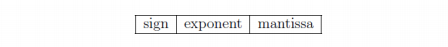Skip to content
Related Articles
GATE | GATE CS 2021 | Set 2 | Question 14
• Last Updated : 23 May, 2021

The format of the single-precision floating point representation of a real number as per the IEEE 754 standard is as follows:Which one of the following choices is correct with respect to the smallest normalized positive number represented using the standard?
(A) exponent =00000000 and mantissa =0000000000000000000000000
(B) exponent =00000000 and mantissa =0000000000000000000000001
(C) exponent =00000001 and mantissa =0000000000000000000000000
(D) exponent =00000001 and mantissa =0000000000000000000000001

Answer: (C)

Explanation: The smallest biased exponent for the normalized number is E=1.

The smallest Mantissa M = 000…0

The smallest positive normalized number
= 1.M x 2^(E-127)
= 1.0 x 2^(-126)
= 2^(-126)
Correct option C.

Attention reader! Don’t stop learning now. Learn all GATE CS concepts with Free Live Classes on our youtube channel.

My Personal Notes arrow_drop_up